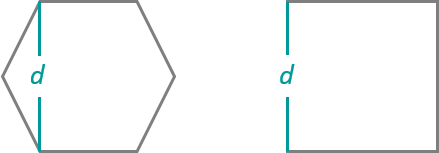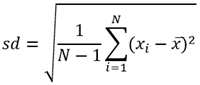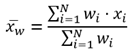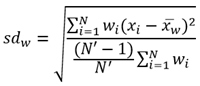# Summarize Within (Map Viewer Classic)

##### Note:

This tool is now available in Map Viewer, the modern map-making tool in ArcGIS Online. To learn more, see Summarize Within (Map Viewer).The Summarize Within tool calculates statistics in areas where an input layer overlaps a boundary layer.

## Workflow diagram## Examples

• A city has a backlog of maintenance projects and wants to involve the maintenance assessment districts in projects related to streetlight and bike route maintenance. The Summarize Within tool is used to count the number of streetlights and miles of bike routes in each district so that the projects can be prioritized effectively.

See the Facility inventory by district case study for the complete workflow.

• A cable provider is starting a pilot program in which it provides low-cost internet access to low-income community college students. The Summarize Within tool is used to determine the number of low-income families in each college district so the cable provider can choose an appropriate district for its pilot program.

See the Districts with low income families case study for the complete workflow.

• A development company is considering creating a mixed-use development in an urban center. The company can use Summarize Within to summarize the qualifying zones within the city's boundary to find the total area of potential development zones.

See the Mixed-use development case study for the complete workflow.

## Usage notes

The inputs for Summarize Within include one area layer that serves as the boundary for summarizing features and a point, line, or area layer to be summarized.

You can provide the area layer to use for analysis, or you can generate bins of a specified size and shape (hexagon or square) into which to aggregate. The bin size specifies how large the bins are. If you are aggregating into hexagons, the size is the height of each hexagon and the width of the resulting hexagon will be 2 times the height divided by the square root of 3. If you are aggregating into squares, the bin size is the height of the square, which is equal to the width.##### Tip:

You can add a layer that is not in Map Viewer Classic to the tool pane by selecting Choose Analysis Layer on the drop-down menu.

A Count of Points, Length of Lines, or Sum Area check box appears depending on the type of features to summarize in your layer. The box is checked by default and can only be unchecked if statistics are being calculated. The default distance measure depends on the units in your profile.

CalculationInput featuresDefaultOptions

Count of Points

Points

None

None

Length of Lines

Lines

Miles (U.S. Standard setting) or Kilometers (Metric setting)

• Miles
• Feet
• Kilometers
• Meters
• Yards

Sum Area

Areas

Square Miles (U.S. Standard setting) or Square Kilometers (Metric setting)

• Square Miles
• Square Kilometers
• Square Meters
• Hectares
• Acres

There are five options for statistics that you can calculate on numeric fields in the layer to be summarized: sum, minimum, maximum, average, and standard deviation. Each time a Field and Statistic are entered, a new row is added to the tool pane so that more than one statistic can be calculated at once. You can view the summarized data in the result layer's table or pop-ups.

Optionally, you can select a group by field so that statistics are calculated separately for each unique attribute value. When a group by field is selected, the pop-up for each feature in the output layer contains charts showing each summary total and statistic by field value. A summary table listing each feature and statistic by group by field value is also created.

The Add minority, majority and Add percentages check boxes are enabled when a group by field is entered. The minority and majority values are the least and most dominant values, respectively, from the group by field, where dominance is determined using the count of points, total length, or total area of each value. When Add minority, majority is checked, two new fields are added to the result layer. The fields list the values from the group by field that are the minority and majority for each result feature. When Add percentages is checked, a new field is added to the result table listing the percentage of the count of points, total length, or total area within each feature that belongs to each value from the group by field. If Add minority, majority is also checked, two additional fields are added to the result layer listing the percentage of the count of points, total length, or total area that belongs to the minority and majority values for each feature.

If Use current map extent is checked, only those features in the input layer and the layer to be summarized that are visible within the current map extent will be analyzed. If unchecked, all features in both the input layer and the layer to be summarized will be analyzed, even if they are outside the current map extent.

##### Tip:

Click Show Credits before you run your analysis to check how many credits will be consumed.

## Limitations

• The boundary input must be area features.
• Lines and areas are summarized using proportions; it is best to summarize absolute data (such as population) rather than relative data (such as average income) when lines or areas are being summarized.
• There is no option to remove boundaries that do not overlap any points, lines, or areas.

## How Summarize Within works

The sections below describe the functionality of the Summarize Within tool.

### Generating bins

Square and hexagonal bins can be generated for aggregation areas rather than summarizing features into an input area layer. The bins are generated in a custom, area-preserving projected coordinate system using the specified size dimensions to ensure the sizes are equal and appropriate for the area of interest. An appropriate equal-area projection and parameters are chosen based on the geographic extent of the input layers. Once bins are created, they are projected back to the coordinate system of the input data before being used in the analysis.

After the analysis is complete, the result is projected to Web Mercator for display (the default) or to the projection of your custom basemap. A Web Mercator projection may cause your results to appear distorted, especially for large bins or bins near the polar regions. These distortions are part of the display only and do not reflect an inaccurate analysis.

### Equations

Average and Std Deviation are calculated using weighted mean and weighted standard deviation for line and area features. None of the statistics for point features are weighted. The following table shows the equations used to calculate standard deviation, weighted mean, and weighted standard deviation:

StatisticEquationVariablesFeatures

Standard Deviationwhere:

• N = Number of observations
• xi = Observations
• = Mean

Points

Weighted Meanwhere:

• N = Number of observations
• xi = Observations
• wi = Weights

Lines and Areas

Weighted Standard Deviationwhere:

• N = Number of observations
• xi = Observations
• wi = Weights
• w = Weighted mean
• N' = Number of non-zero weights

Lines and Areas

##### Note:

Null values are excluded from all statistical calculations. For example, the mean of 10, 5, and a null value is 7.5 ((10+5)/2).

### Points

Point layers are summarized using only the point features within the input boundary. The number of points that are within each input boundary is only included in the results if the Count of Points box is checked. The results are displayed using graduated symbols.

The figure and table below show the statistical calculations of a point layer within a hypothetical boundary. The Population field was used to calculate the statistics (Sum, Minimum, Maximum, Average, and Std Deviation) for the layer.Point layers are summarized using only points located within the boundary layer. An example attribute table is displayed above with values to be used in hypothetical statistic calculations.
StatisticResults District A

Sum

``280 + 408 + 356 + 361 + 450 + 713 = 2,568``

Minimum

Minimum of:

``[280, 408, 356, 361, 450, 713] = 280``

Maximum

Maximum of:

``[280, 408, 356, 361, 450, 713] = 713``

Average

``2,568/6 = 428``

Std Deviation``= 150.79``

A real-life scenario in which you can use this analysis is determining the total number of students in each school district. Each point represents a school. The Type field contains the type of school (elementary, middle school, or secondary) and a student population field contains the number of students enrolled at each school. The calculations and results are provided for District A in the table above. From the results, you can see that District A has 2,568 students. When running the Summarize Within tool, the results are also provided for District B.

### Lines

Line layers are summarized using only the proportions of the line features that are within the input boundary. When summarizing lines, use fields with counts and amounts rather than rates or ratios so proportional calculations make logical sense in your analysis. The results include the number of lines that are within each input boundary and are displayed using graduated symbols.

The figure and table below show the statistical calculations of a line layer within a hypothetical boundary. The Volume field was used to calculate the statistics (Sum, Minimum, Maximum, Average, and Std Deviation) for the layer. The statistics are calculated using only the proportion of the lines that are within the boundary.Line layers are summarized using only the proportions of the lines located within the boundary.
StatisticResult

Sum

``400 + 500 = 900``

Minimum

Minimum of:

``[400, 500] = 400``

Maximum

Maximum of:

``[400, 500] = 500``

Average``= 460``

Std Deviation``= 69.28``

A real-life scenario in which you can use this analysis is determining the total volume of water in rivers within the boundaries of a state park. Each line represents a river that is partially located inside the park. From the results, you can see that there are 5 miles of rivers within the park and the total volume is 900 units.

### Areas

Area layers are summarized using only the proportions of the area features that are within the input boundary. When summarizing areas, use fields with counts and amounts rather than rates or ratios so proportional calculations make logical sense in your analysis. The results include the number of areas that are within each input boundary and are displayed using graduated colors.

The figure and table below show the statistical calculations of an area layer within a hypothetical boundary. The populations were used to calculate the statistics (Sum, Minimum, Maximum, Average, and Std Deviation) for the layer. The statistics are calculated using only the proportion of the area that is within the boundary.Area layers are summarized using only the proportions of the areas located within the boundary.
StatisticResult

Sum

``2,133 + 3,133 + 400 + 3,375 + 1,800 = 10,841``

Minimum

Minimum of:

``[2,133, 3,133, 400, 3,375, 1,800] = 400``

Maximum

Maximum of:

``[2,133, 3,133, 400, 3,375, 1,800] = 3,375``

Average``= 2,665.53``

Std Deviation``= 925.91``

A real-life scenario in which you can use this analysis is determining the population in a city neighborhood. The blue outline represents the boundary of the neighborhood and the smaller areas represent census blocks. From the results, you can see that there are 10,841 people in the neighborhood and an average of approximately 2,666 people per census block.

## Similar tools

Use Summarize Within to calculate statistics for features that overlap a boundary layer. Other tools may be useful in solving similar but slightly different problems.

### Map Viewer Classic analysis tools

If you are summarizing points and want to keep only the boundaries with a count greater than zero, use the Aggregate Points tool.

If you are summarizing features within a distance of your input features, use the Summarize Nearby tool.

### ArcGIS Pro analysis tools

Summarize Within performs the functions of the Spatial Join and Summary Statistics geoprocessing tools.